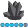jonatan_velarde
Pyrite | Level 9

## How to name decimal characters in SAS - Loop for data set

Good day SAS friends:

I need to know how to name this variable in the example, im working in categorical variables, so when the sample has no decimal characters, this means succes ("1") and when the characters have decimal characters means no succes ("0").

here is the data set and my programming (help me to fix it please):

data main;
input sample;
cards;
23

24

25

26.7

28

28.1

28.2

28.3
;
run;

data want;

set have;
z = .;

if sample = (number with no decimals)

then do;

z = 1;

result = "Succes!";
end;
else if x = (number with decimals) then do;

z = 0;

result = "No Succes";
end;
run;

proc print data=want;
var sample z result;
run;

Thank you very much

1 ACCEPTED SOLUTION

Accepted Solutions

## Re: How to name decimal characters in SAS - Loop for data set

If your data came in as numeric as you presented:

```data main;
input sample;
cards;
23
24
25
26.7
28
28.1
28.2
28.3
;
run;```

data want;

set main;

length result \$ 10;

z=sample=int(sample);

result=ifc(z=1,'success', 'no success');

run;

3 REPLIES 3

## Re: How to name decimal characters in SAS - Loop for data set

``````data want;
set main;
z=0;
result='No Succes';
if index(sample,'.') then do;
z = 1;
result = "Succes!";
end;
run;``````

## Re: How to name decimal characters in SAS - Loop for data set

If your data came in as numeric as you presented:

```data main;
input sample;
cards;
23
24
25
26.7
28
28.1
28.2
28.3
;
run;```

data want;

set main;

length result \$ 10;

z=sample=int(sample);

result=ifc(z=1,'success', 'no success');

run;jonatan_velarde
Pyrite | Level 9

## Re: How to name decimal characters in SAS - Loop for data set

Awesome

Thank a lot !!!

Discussion stats
• 3 replies
• 1310 views
• 0 likes
• 3 in conversation